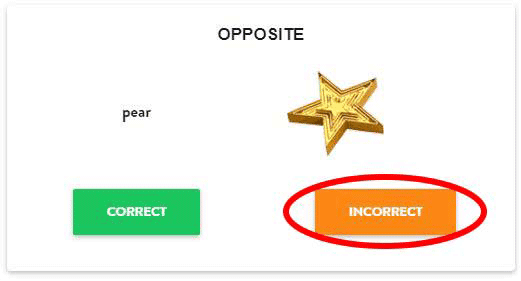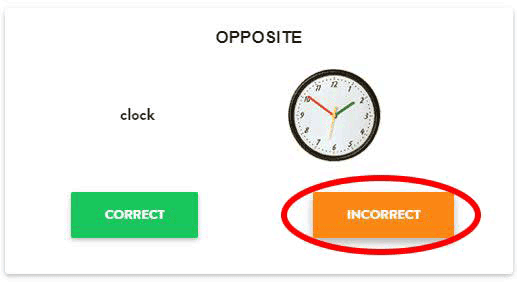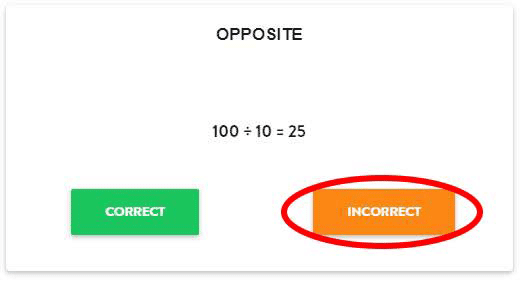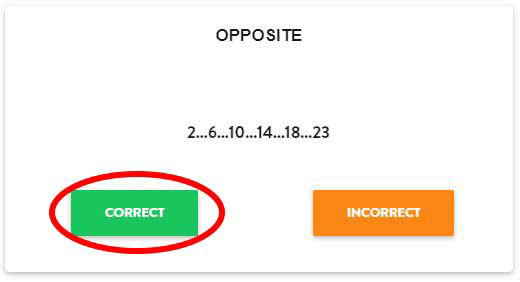### Mental Speed Test

•  Number of questions:  40
•  Test duration:  5 minThe following test is meant to assess your mental speed - how quickly you can process information and make decisions based upon that information. The exercise consists of word/image pairs and simple mathematical equations or number sequences. If a pair matches, click the "Correct" button (the left arrow key on your keyboard). If the pair does not match, click the "Incorrect" button (the right arrow key on your keyboard). However, if the word "Opposite" appears at the top of the screen, you need to reverse your answer.In the first example (pear and star), the answer would be incorrect ("Incorrect"). For the second example, although it is an exact match, the word Opposite appears at the top of the screen, so rather than choosing "Correct" you would have to choose "Incorrect"

For the mathematical equations simply indicate whether the answer is correct or incorrect. For the number sequences indicate whether the number in red correctly completes the sequence.As you can see, the first equation is wrong - the answer should be 5, so in this case, you would choose "incorrect". However, although the number sequence is also wrong (the answer should be 22), the word Opposite requires you to reverse your answer, so in this case you would choose "Correct" rather than "Incorrect".

Remember, you are being timed, so try to answer as quickly as possible - and remember to reverse your answer when the word "Opposite" appears.

After finishing the test, you will find out how accurate and fast you were. Have fun!